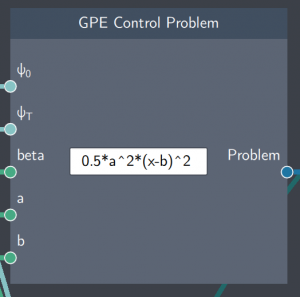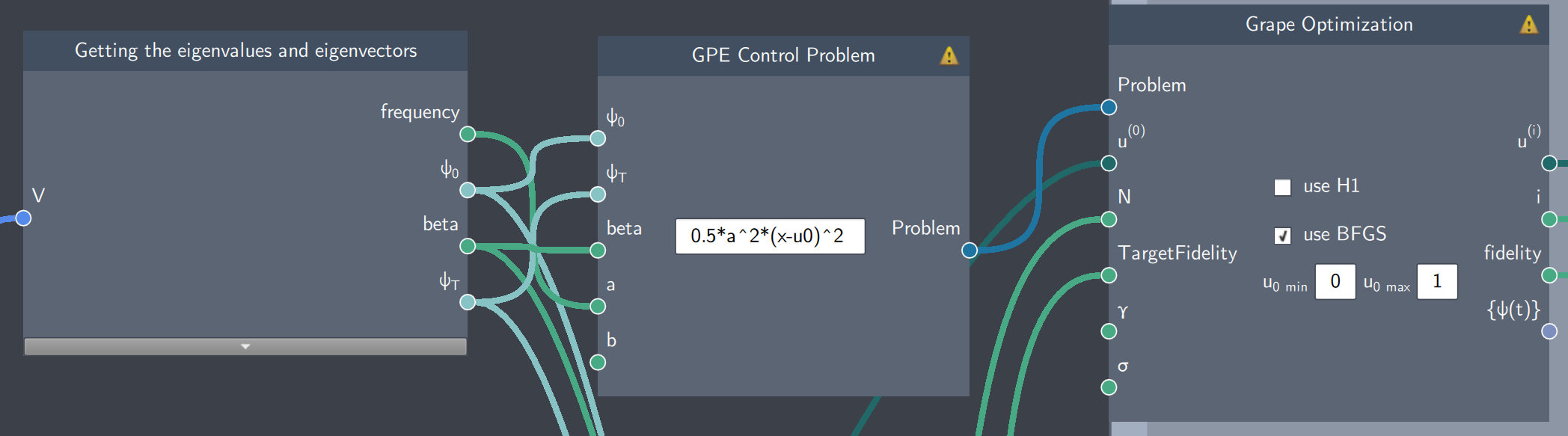# GPE Control Problem

## Description

The GPE Control Problem node lets you define Bose-Einstein Condensate (BEC) quantum optimal control problems.## Inputs

The node has the following inputs:

• Initial state ($\psi_{o}$): The initial state of the control problem
• Target state ($\psi_{T}$): The target state of the control problem
• Beta: A real scalar value determining the BEC interaction strength
• Scalars (a, b): Any scalar values that parameterize the potential or/and the control problem

## Content

The content field of the node consists of an analytical expression that parameterizes a potential by a control function.

## Output:

• Problem: It outputs the control problem which is used as an input for optimization

## Example

In the example below, a state transfer problem is simulated where a BEC (trapped in a harmonic oscillator potential) is transported from one location to another. The state transfer between an initial and target state is carried out by controlling the potential. In the Control Problem node, the scalar $a$ refers to the frequency of the potential and $u0$  refers to controlling the potential between the two locations.

For another example, you can look at the shake-up optimal control challenge exercise where you can find the details along with the flowscene.